# GMAT Tricks with Systems of Equations: Part 2

A lot of GMAT test-takers vaguely remember a rule from high school, that it’s possible to solve for two variables if and only if you’re given two equations, and generally that it’s possible to solve for n variables if and only if you’re given n equations. Applying this rule incorrectly causes quite a few errors on the quant section, particularly with Data Sufficiency questions.

This is the second post in our series on avoiding those errors:

## Let’s get the rule right!

Take a minute or two to answer this problem:

What is the value of ?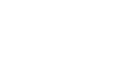(A) Statement (1) ALONE is sufficient, but statement (2) alone is not sufficient.
(B) Statement (2) ALONE is sufficient, but statement (1) alone is not sufficient.
(C) BOTH statements TOGETHER are sufficient, but NEITHER statement ALONE is sufficient.
(D) EACH statement ALONE is sufficient.
(E) Statements (1) and (2) TOGETHER are NOT sufficient.

If we too hastily apply the rule described above, we’ll probably choose (C) right away. After all, x  and y are two variables and (1) and (2) are two equations.  However, the correct answer is (E).

Why? Well, let’s try to solve the problem as we would a system of linear equations and see what happens. We could solve this by simultaneous solution, but I’m going to take the apparently simpler route of substitution, solving for x in terms of y, and then substituting the y-expression for x.

Begin with the first equation.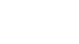Transpose to isolate x.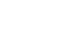Substitute the right-hand expression for x in the second equation.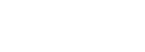Distribute 2 to clear the parentheses.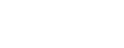Multiply both sides of the equation by y to clear the fraction.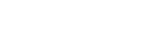Combine like terms and put everything on the left-hand side of the equation.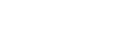Because this is a quadratic but not a quadratic square (not of the Form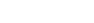). For a Data Sufficiency question we don’t really care what exactly those solutions are, just that there are two of them, and that if we determine the two solutions and the x values that correspond to each, we’ll have two different answers to the question “What is the value of x – y ?” even when we consider both statements, so the answer is (E).

It turns out that the often-vaguely-remembered rule I cited above isn’t quite right. The rule isn’t actually that it’s possible to solve for two variables if and only if you’re given two equations.

## The correct rule

The correct rule is that a system with the same number of distinct linear equations and unknowns has a single unique solution. So if the equations are distinct or aren’t linear, then the rule doesn’t apply.

In this case, the first equation wasn’t linear. That is, it wouldn’t yield a straight line if it were plotted on a coordinate plane. When an equation includes any of the following, it is not linear:

• a variable raised to a power
• wo or more variables multiplied together
• a variable in the denominator of a fraction and a variable in the numerator of another fraction or outside any fraction.

(This is a little oversimplified for the wide world of math, but it’s adequate for the GMAT.)

Notice that in our problem the equation in Statement (1) has one variable (x) in a denominator and another variable (y) outside of any fraction. When we cleared the fraction we found the product xy.

When you are given an equation that is obviously exponential or quadratic, the question might or might not be tricky. But when you are given a question that includes a disguised exponential or quadratic, such that you only discover the complication when you manipulate the equation or combine two equations, then it’s tricky.

Often the answer to such a question will appear to be (C) but will turn out to be (E), as with our problem above. However, there are other possibilities. We’ll consider some of those in my next blog post.

## Author

•Michael Schwartz is really good at standardized tests. He’s earned multiple perfect scores on the GRE, GMAT, and LSAT. He’d rather have perfect pitch or be able to run low 1:40s for the 800 meters, but you take what you get. He has decades of teaching and curriculum-development experience. One of these days he might finish his dissertation and collect that Ph.D. in philosophy. Might.